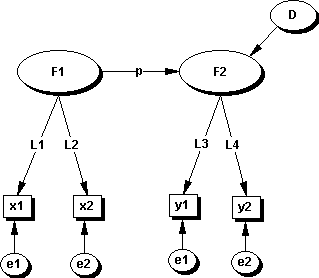Structural Equation Modeling in IS Research - Understanding the LISREL and PLS perspective

Wynne W. Chin

University of Houston

As in many other social science areas, the IS field has seen a substantial increase in the number of submissions and publications using structural equation modeling (SEM) techniques. This is likely due to the proliferation of software packages to perform covariance-based (e.g., LISREL, EQS, AMOS, SEPATH, RAMONA, MX, and CALIS) and component-based (e.g., PLS-PC, PLS-Graph) analysis. The SEM approach is integrative in the sense that it combines the perspective of two research traditions:

1. an econometric perspective focusing on prediction and,
2. a psychometric emphasis that models concepts as latent (unobserved) variables that are indirectly inferred from multiple observed measures (alternately termed as indicators or manifest variables).
This resulting combination allows researchers to perform path analytic modeling with latent variables (LVs). Specifically, SEM provides the researcher with the flexibility to: (a) model relationships among multiple predictor and criterion variables, (b) construct unobservable LVs, (c) model errors in measurements for observed variables, and (d) statistically test a priori substantive/theoretical and measurement assumptions against empirical data (i.e., confirmatory analysis).

SEM involves generalizations and extensions of earlier first-generation procedures. By applying certain constraints or assumptions on an SEM analysis, a researcher can end up performing the equivalent of techniques such as canonical correlation, multiple regression, multiple discriminant analysis, analysis of variance or covariance, or principle components analysis.

Naturally, along with the benefits comes the complexity. This virtual discussion will cover various issues that often appear among researchers. The primary focus will be on both the covariance based approach often equated generically as a LISREL analysis and the Partial Least Squares approach. Hopefully, we will not only cover matters generic to social scientists, but also specific to the IS field. To guide the questions, we might look at it from various frames.

One standard approach is to examine the stages in the traditional SEM lifecycle. They are:

• Model Specification,

•
• Identification,

•
• Estimation,

•
• Testing Fit, and

•
• Model Modification or Respecification.
Another approach is to examine common mistakes that are made. We can discuss such issues as:

• Critical Missing Information. Information that should be included but are often left out from research articles thereby preventing other researcher from reproducing the analysis and building a cumulative tradition.

•
• Mismatch of questionnaire items and subsequent analysis. Survey questions analyzed are often formative in nature or a composite of formative and reflective measures. A LISREL analysis and use of internal consistency measures such as Cronbachs alpha would be incorrect.

•
• Sole reliance of overall goodness of fit measures. Using only covariance based goodness of fit measures as the primary arbiter of confirmation while ignoring other important measures of model adequacy.

•
• Analyzing second order factors without a purpose. Demonstrating second order factor models without providing an underlying rationale for its subsequent usage.

•
• Lack of Empirical over-identification. Many empirical studies do not perform a strong test of the model/latent variables.

•
• Ignoring the statistical power of models.

•
• Ignoring equivalent models.

•
• Falling into an exploratory mode via initial exploratory factor analysis or using information from the statistical package to modify initial models for better fit.

•
• Premature or inappropriate approach of analysis when either substantive or theoretical knowledge is relatively new.

Finally, we can approach it from an applied perspective. Example questions might include:

• When should I consider using Partial Least Squares as opposed to LISREL?

•
• More importantly - how does Partial Least Square differ from LISREL?

•
• How does LISREL compare to path analysis using multiple regression?

•
• Does it make sense to do an exploratory factor analysis prior to using SEM?

•
• How about a confirmatory factor analysis first?

•
• What are the advantages to using SEM for multi-sample or cross-cultural analysis?
To start off, we might consider the following model (Figure 1) as a basis for discussion. The model is a simple two factor model where F1 is hypothesized to affect F2. The data in the form of a correlation matrix is provided in Table 1 for the four measures/indicators (x1,x2,y1,and y2) of their respective factors.Figure 1. Two factor model with two indicator/measures for each factor.

 x1 x2 y1 y2 x1 1.00 x2 .087 1.00 y1 .140 .080 1.00 y2 .152 .143 .272 1.00

Table 1. Sample Data Set (n=1000).

All correlations, given the sample size, are significant but quite low ranging from 0.087 to 0.272. If we use the theoretical model as depicted in Figure 1, what would be the path estimate p linking F1 and F2? The covariance based estimate using software such as LISREL would result in a standardized estimate of p at 0.83 whereas the PLS estimate was 0.22. The standardized loadings of a, b, c, d using the covariance procedure were 0.33, 0.26, 0.46, and 0.59. The PLS estimates resulted in loadings of 0.81, 0.66, 0.73, and 0.85 with corresponding weights of 0.75, 0.60, 0.54, and 0.71. In the case of the covariance estimates, the path estimate of 0.83 is much larger than the observed correlations between the x and y variables where the highest is 0.152. In the case of PLS, the estimate of 0.22 is much closer to the observed correlations. Which estimate should we place confidence in?